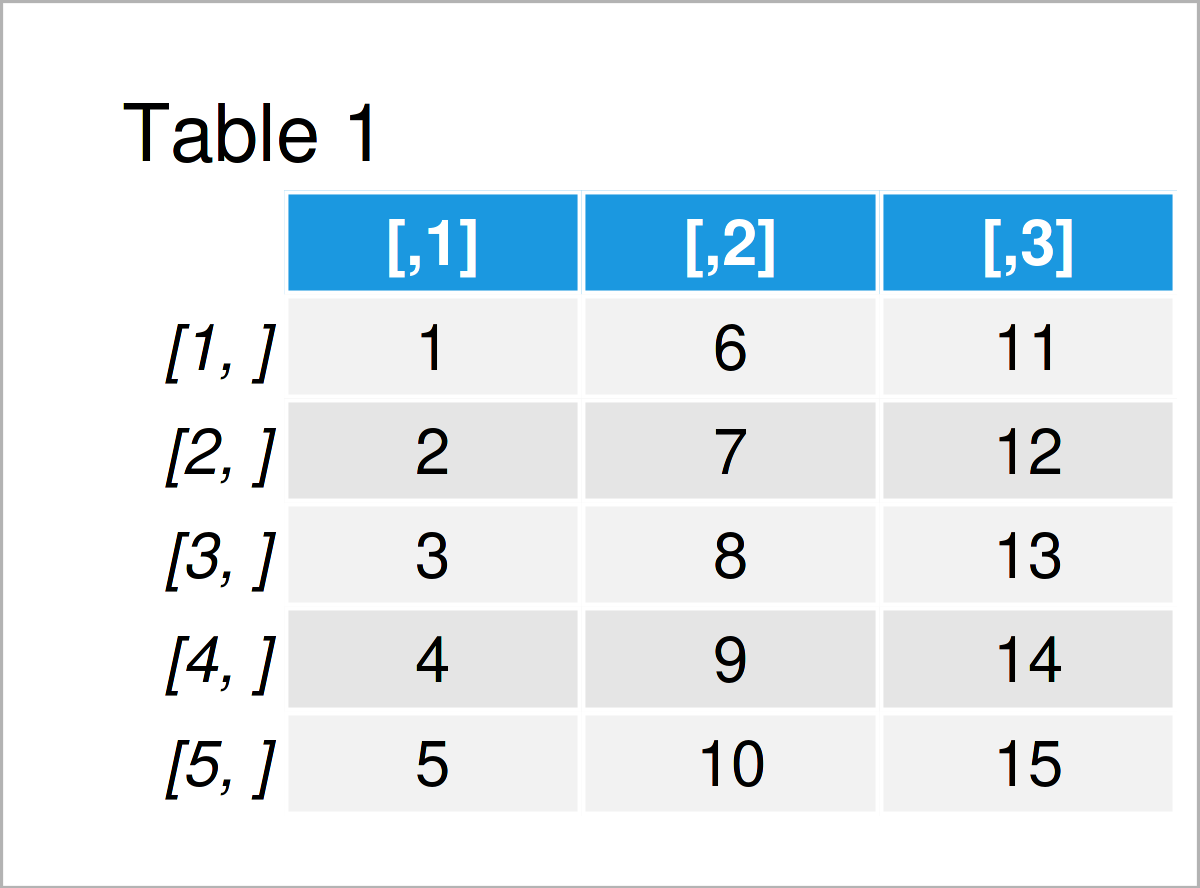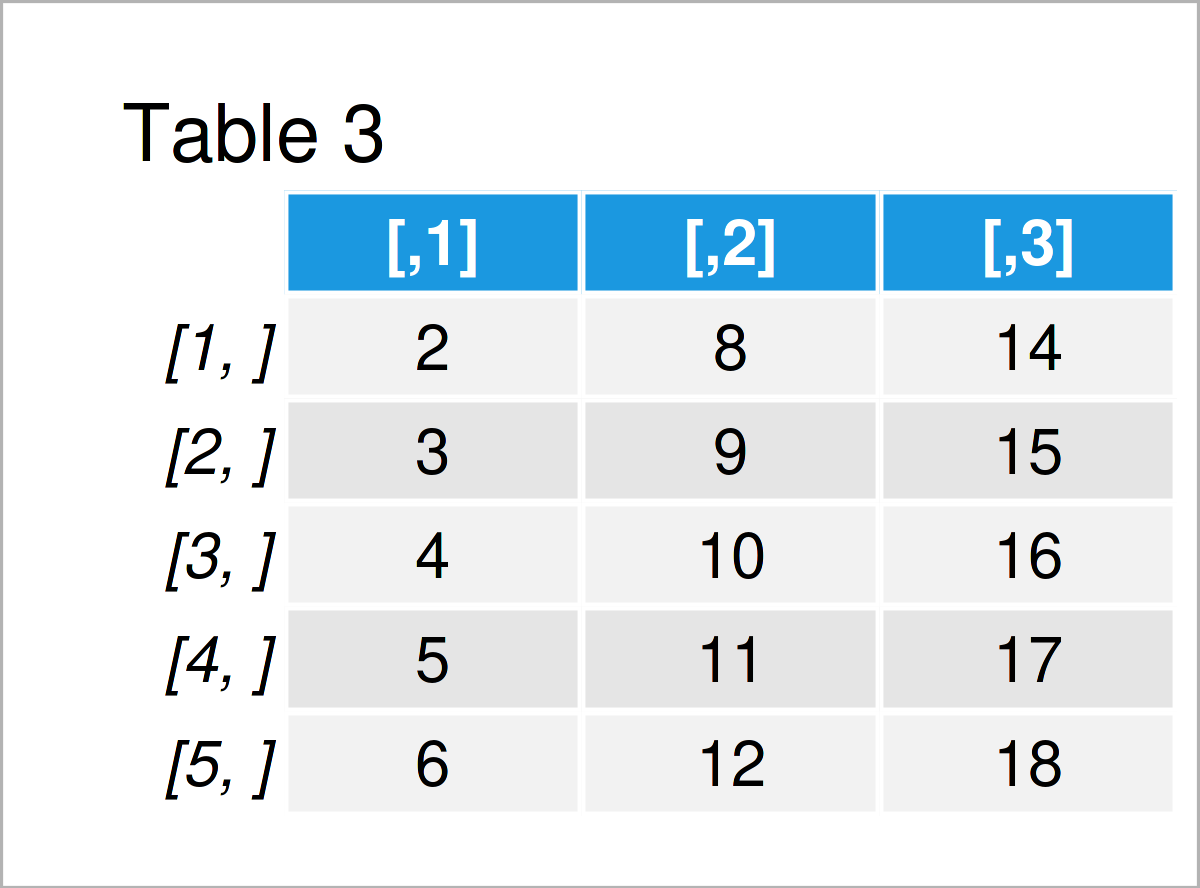# How to Subtract / Add a Vector from / to Each Row of a Matrix in R (2 Examples)

In this article, you’ll learn how to subtract or add a constant vector from each row of a matrix in the R programming language.

The tutorial will consist of two examples for the subtraction or addition of the values in a vector from each row of a matrix. More precisely, the page looks as follows:

Here’s how to do it.

## Creation of Example Data

We’ll use the following data as basement for this R programming tutorial:

```my_mat <- matrix(1:15, ncol = 3)    # Create example matrix
my_mat                              # Print example matrix```Table 1 shows the structure of our example data: It consists of five rows and three columns.

Next, we also have to create a vector object that we can subtract and add from / to the cells of our data set:

```my_vec <- 1:3                       # Create example vector
my_vec                              # Print example vector
#  1 2 3```

Our vector contains the values 1, 2, and 3.

## Example 1: Subtract Vector from Each Row of Matrix Using sweep() Function

Example 1 explains how to subtract constant values from every row of a matrix in R.

For this task, we can use the sweep function as explained below:

```my_mat_new1 <- sweep(my_mat,        # Apply sweep function
2,
my_vec)
my_mat_new1                         # Return updated matrix```Table 2 illustrates the output of the previous R programming syntax: We have created a new matrix object where our example vector has been subtracted from our example matrix.

## Example 2: Add Vector to Each Row of Matrix Using sweep() Function

The following syntax demonstrates how to add values in a vector to each row of a matrix or data frame.

Again, we can use the sweep function. However, this time we have to specify the FUN argument to be equal to “+”.

```my_mat_new2 <- sweep(my_mat,        # Apply sweep function
2,
my_vec,
FUN = "+")
my_mat_new2                         # Return updated matrix```In Table 3 it is shown that we have created another output matrix where the constant numbers in our vector have been added to the values in our input matrix.

## Video, Further Resources & Summary

In case you need further info on the examples of this tutorial, you might want to have a look at the following video on my YouTube channel. In the video, I’m explaining the R programming syntax of the present article:

In addition to the video, you might want to have a look at some of the related tutorials on this website.

In this article, I have shown how to subtract or add a vector from each row of a matrix object in the R programming language. Let me know in the comments section below, in case you have further questions.

Subscribe to the Statistics Globe Newsletter